# Statistical Measures of Central TendencyPhoto by erika m on Unsplash

# Introduction

In statistics, measures of central tendency are a set of “middle” values representative of the data points. Central tendency describes the distribution of data focusing on the central location around which all other data are clustered. It is the opposite of dispersion that measures how far the observations are scattered with respect to the central value.

As we will see below, central tendency is an elementary statistical concept, yet a widely used one. Among the measures of central tendency mean, median and mode are most frequently cited and used. Below we will see why they are important in the field of data science and analytics.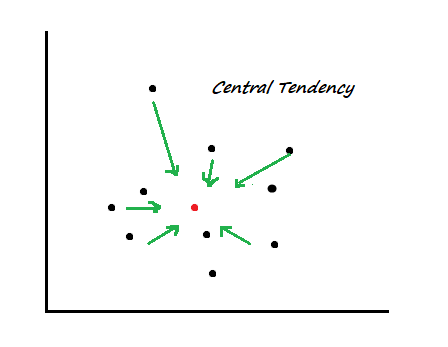Figure: Conceptualizing measures of Central Tendency

# 1. Arithmetic Mean

Mean is the average of some data points. It is the simplest measure of central tendency that takes the sum of the observations and divides the sum by the number of observations.

In mathematical notation arithmetic mean is expressed as: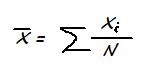Where xi are individual observations and N is the number of observations

In a more practical example, if wages of 3 restaurant employees are \$12, \$14 and \$15 per hour, then the average wage is \$13.6 per hour. Simple as that.

Application of mean
• We do all kinds of averages in our everyday life. We ask friends about average house rents in their neighborhoods; we calculate monthly expenses before moving to a new city. We use the arithmetic mean every day, in all contexts.
• Businesses use means to compare the average daily sales of a product between January and February.
• In data science, mean is an essential metric in exploratory data analysis (EDA) and is an input to all kinds of advanced modeling. Mean works behind the scene in calculating RMSE (Root Mean Squared Error), MAE (Mean Absolute Error) accuracy metrics in classification or regression algorithms.

Typology

There are a few variants of the mean. Those are not used as frequently but are useful tools in specialized use cases. Below are some examples:

Weighted mean
In ordinary mean, all data points are treated equally and equal weights are allocated (implicitly) to all data points. In weighted mean, some data are given higher (or lower) weights depending on the objectives.

Geometric mean
Unlike ordinary mean, geometric mean multiplies N values and take N-th root of the product. So, for two values 2 & 8, the geometric mean would be 4.

Harmonic mean
It is another kind of mean that is calculated by taking reciprocals of data points, then taking their overage and finally taking reciprocal of the result.

Limitations of mean

Although arithmetic means is the most widely known measure of Central Tendency, it is not a robust metric; it can be highly sensitive to outliers.

Let’s consider the following two cases. On the left, the average of the four values is perfectly in the middle of the dataset. However, on the right, just one outlier data (16) changed the “center of gravity” and dragged the mean to further right. To overcome this limitation of arithmetic mean, we have another measure of central tendency — Median.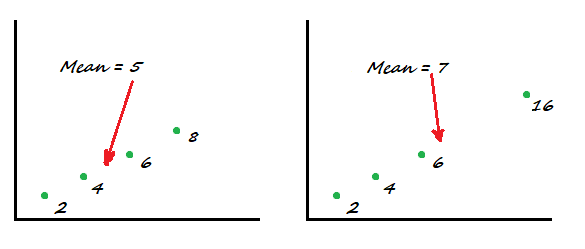Figure: Impact of an outlier on the arithmetic mean of a dataset (illustration: author)

# 2. Median

What number is at the center of the list [2, 3, 4]? The answer is of course 3. And that’s the median. What if the same numbers are ordered differently, say [2, 4, 3]? Is the median now 4? No, it’s still 3. So median is the number at the center of a series after they are ordered (ascending or descending).

Let’s say we have a list of five numbers [4, 6, 2, 10, 7] and we want to find the median. The process is simple:
1. Data: [4, 6, 2, 10, 7]
2. Order the list: [2, 4, 6, 7, 10]
3. Find the number at the center: 6 (median)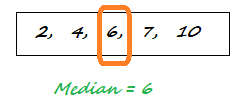But what if we have even numbers in the list [4, 7, 6, 2, 10, 8]? Now there are two values in the middle, so in this case the solution is to take an average of them:
1. Data: [4, 7, 6, 2, 10, 8]
2. Order the list: [2, 4, 6, 7, 8, 10]
3. Find two numbers at the center: [6, 7]
4. Take an average: 6.5 (median)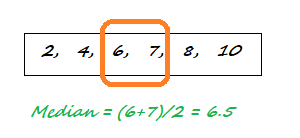Why median and what’s the benefit of using it as a measure of central tendency? One big reason is, unlike mean, it’s not sensitive to extreme values. For example in the list [2, 3, 4] the last value could have been 400 instead of 4, yet the median will remain the same 3.

The other good case for median is the interpretation of data. Median splits data perfectly into two halves, so if median income in Howard County is \$100,000 per year, you could simply say that half the population has higher and the remaining half has lower than \$100k income in the county.

However, there is an obvious disadvantage. Median uses the position of data points rather than their values. That way some valuable information is lost and we have to rely on other kinds of measures such as measures of dispersion (next section) to get more information about the data.

Use cases

Some applications of median are well-known. Have you noticed that the US Census Bureau reports household income as “Median household income”? Or Bureau of Labor Statistics reporting wages of Americans as “Median wage”? That is because the large number of data collected through surveys or census are highly dispersed having both extremely small and large values. In such cases, median is a better measure of the center of distributions than mean is.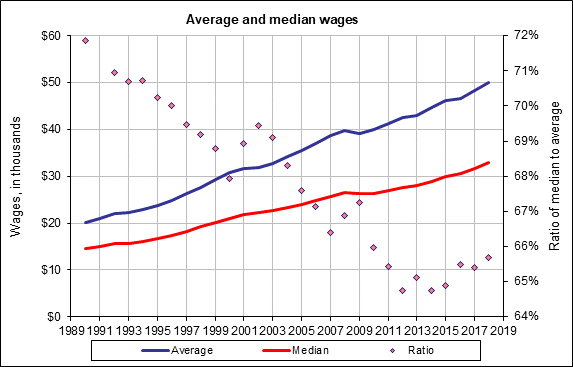Figure: Average and median wages in the US. (
Source: Social Security Administration; accessed: July 19, 2020)

# 3. Mode

In a list of numbers — say, [2, 3, 4, 4] — the most frequently occurring one is 4; that’s mode. It can apply to text data as well, for example, the mode of the set {“John”, “Kelly”, “Nadia”, “John”, “Michael”} is “John”, because it appears twice in this set of names.

A distribution can have more than one mode as in the list [2, 2, 3, 4, 4]; it’s called bimodal distribution of a discrete variable. Along this logic, a distribution with more than two modes are called multimodal distribution.

Use cases
• Understanding mode of a distribution is important because frequently occurring values are more likely to be picked up in a random sample.
• What’s the most frequently occurring first name in a city? Mode has the answer. Understanding mode helps with many more such problems in the field of Natural Language Processing (NLP).
• Mode can help a grocery chain figure out which product is selling the most on different days of the week, month or year.

# Summary

In summary, central tendency is an important set of concepts in statistics and data science that measures how some observations are positioned around a central value. Arithmetic mean is simply an average of datapoints, median is the value at the center of a dataset and mode returns the most frequently occurring value (numeric or text). These measures have a wide number of use cases in data science — from exploratory data analysis to measuring accuracy metrics in classification algorithms to natural language processing.

“Statistical Measures of Central Tendency”
– Mahbubul Alam

1.ds1
over 1 year ago
central tendency :) always a hard topic for me

### Related Stories

Nov 25, 2021

#### 5 Tips To Ace Your Job Interview For A Data Scientist Opening

5 Tips To Ace Your Job Interview For A Data Scientist Opening.PNG 795.94 KBImage SourceAspiring data scientists have a bright future ahead of them....By Daniel Morales
Nov 12, 2021

#### When to Avoid Deep LearningBy Matt Przybyla
Oct 16, 2021

#### 6 Advanced Statistical Concepts in Data Science

The article contains some of the most commonly used advanced statistical concepts along with their Python implementation.In my previous articles Be...By Nagesh Singh Chauhan